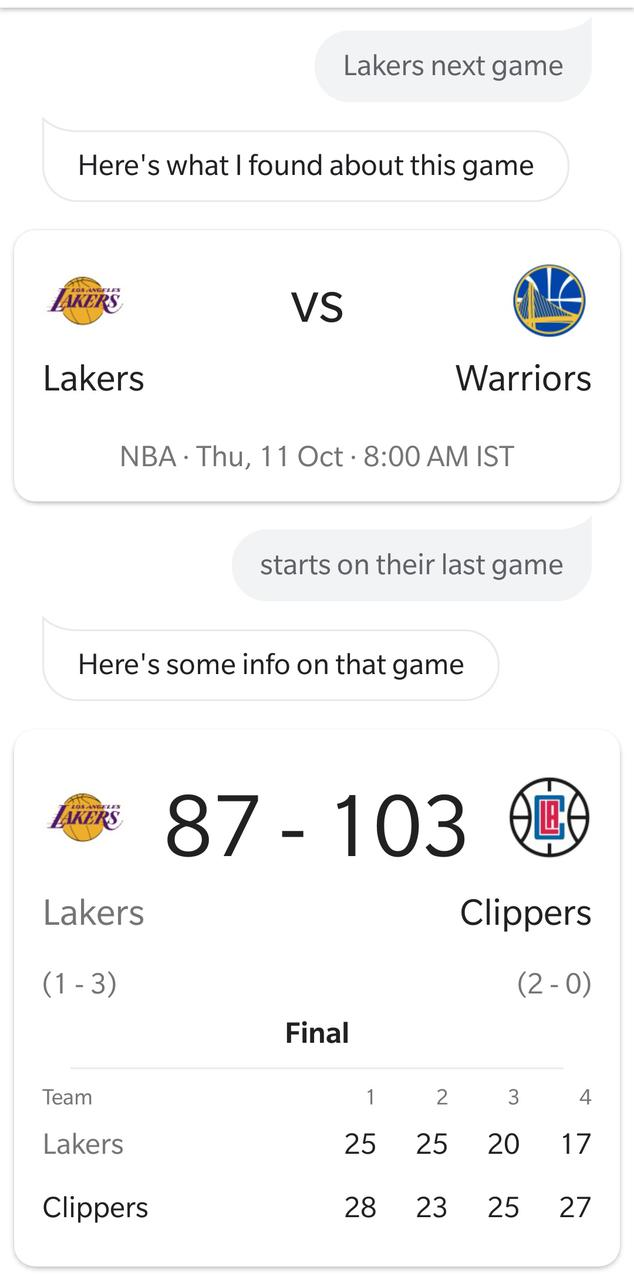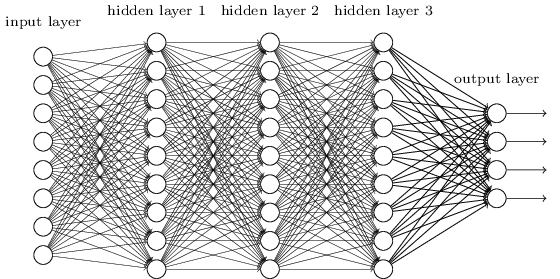### dhruv's space

This is the homepage and blog of Dhruv Thakur, a Data Scientist in the making. Here's what I'm up to currently. For more about me, see here.

# Understanding ResNets

I'm currently enrolled in fastai's Deep Learning MOOC (version 3), and loving it so far. It's only been 2 lectures as of today, but folks are already building awesome stuff based on the content taught so far.

The course starts with the application of DL in Computer Vision, and in the very first lecture, course instructor Jeremy teaches us how to leverage transfer learning by making use of pre-trained ResNet models. I've been meaning to dive into the details of Resnets for a while, and this seems like a good time to do so.

This post is written in the vein of a summary-note, rather than that of a full-fledged introduction to resnets, ie, it's (sort-of) written for my own future reference, and can be helpful for somebody with some background on the topic.

# Word Embeddings and RNNs

One of the simplest ways to convert words from a natural language into mathematical tensors is to simply represent them as one-hot vectors where the length of these vectors is equal to the size of the vocabulary from where these words are fetched.

For example, if we have a vocabulary of size 8 containing the words:

"a", "apple", "has", "matrix", "pineapple", "python", "the", "you"

the word "matrix" can be represented as: [0,0,0,1,0,0,0,0]

Obviously, this approach will become a pain when we have a huge vocabulary of words (say millions), and have to train models with these representations as inputs. But apart from this issue, another problem with this approach is that there is no built-in mechanism to convey semantic similarity of words. eg. in the above example, apple and pineapple can be considered to be similar (as both are fruits), but their vector representations don't convey that.

Word Embeddings let us represent words or phrases as vectors of real numbers, where these vectors actually retain the semantic relationships between the original words. Instead of representing words as one-hot vectors, word embeddings map words to a continuous vector space with a much lower dimension.

# Summary Notes: GRU and LSTMs

This post is sort-of a continuation to my last post, which was a Summary Note on the workings of basic Recurrent Neural Networks. As I mentioned in that post, I've been learning about the workings of RNNs for the past few days, and how they deal with sequential data, like text. An RNN can be built using either a basic RNN unit (described in the last post), a Gated Recurrent unit, or an LSTM unit. This post will describe how GRUs/LSTMs learn long term dependencies in the data, which is something basic RNN units are not so good at.

# Summary Notes: Basic Recurrent Neural Networks

I've been learning about Recurrent Neural Nets this week, and this post is a "Summary Note" for the same.

A "Summary Note" is just a blog post version of the notes I make for something, primarily for my own reference (if I need to come back to the material in the future). These summary notes won't go into the very foundations of whatever they're about, but rather serve as a quick and practical reference for that particular topic.

RNNs are inherently different from a traditional feed-forward neural nets, in that, they have the capability to make predictions based on past/future data. This ability to sort of "memorise" past/future data is crucial to handling cases which have a temporal aspect to them. Let's take the following conversation between me and Google Assistant on my phone:# Visualizing Convolutions

I'm currently learning about Convolutional Neural Nets from deeplearning.ai, and boy are they really powerful. Some of them even have cool names like Inception Network and make use of algorithms like You Only Look Once (YOLO). That is hilarious and awesome.

This notebook/post is an exercise in trying to visualize the outputs of the various layers in a CNN. Let's get to it.

In :
import numpy as np
import pandas as pd

from math import ceil
import matplotlib.pyplot as plt
import matplotlib.image as mpimg
from sklearn.model_selection import train_test_split

from keras import layers
from keras.layers import Input, Dense, Activation, ZeroPadding2D, Flatten, Conv2D
from keras.layers import AveragePooling2D, MaxPooling2D
from keras.models import Model
from keras.datasets import fashion_mnist
from keras.optimizers import Adam
from keras.models import load_model

/usr/lib/python3/dist-packages/h5py/__init__.py:34: FutureWarning: Conversion of the second argument of issubdtype from float to np.floating is deprecated. In future, it will be treated as np.float64 == np.dtype(float).type.
from ._conv import register_converters as _register_converters
Using TensorFlow backend.


To begin with, I'll use the Fashion-MNIST dataset.

# Visualizing Optimisation Algorithms

The first and second courses by deeplearning.ai offer a great insight into the working of various optimisation algorithms used in Machine Learning. Specifically, they focus on Batch Gradient Descent, Mini-batch Gradient Descent (with and without momentum), and Adam optimisation. Having finished the two courses, I've wanted to go deeper into the world of optimisation. This is probably the first step towards that.

This notebook/post is an introductory level analysis on the workings of these optimisation approaches. The intent is to visually see these algorithms in action, and hopefully see how they're different from each other.

The approach below is greatly inspired by this post by Louis Tiao on optimisation visualizations, and this tutorial on matplotlib animation by Jake VanderPlas.

In :
# imports
import matplotlib.pyplot as plt
import numpy as np
from mpl_toolkits.mplot3d import Axes3D
from matplotlib.colors import LogNorm
from matplotlib import animation
from IPython.display import HTML
import math
from itertools import zip_longest
from sklearn.datasets import make_classification

In :
%matplotlib inline


Alright. We need something to optimize. To begin with, let's try to find the minima(s) of Himmelblau function , which is represented as:

$$f(x,y)=(x^{2}+y-11)^{2}+(x+y^{2}-7)^{2}$$

# Moving from Jekyll to Nikola

For some time I have been looking for ways to incorporate Jupyter notebooks into my blog. I used to blog using Jekyll and while it has great support for code blocks (and otherwise being an awesome blog, it lacks native support for ipynb notebooks.

Using nbconvert to convert a notebook to markdown technically works, but the results aren't always pretty, especially if you have tables (such as pandas dataframe outputs) in your notebooks. You need to add custom CSS for to render tables nicely and I'm not so keen on doing that. One solution I came up with is to convert dataframe outputs to images, which technically works fine, but you need to do that every single time you run a df.head() command.

# Summary Notes: Forward and Back Propagation

I recently completed the first course offered by deeplearning.ai, and found it incredibly educational. Going forwards, I want to keep a summary of the stuff I learn (for my future reference) in the form of notes like this. This one is for forward and back-prop intuitions.

### Setup:

A neural network with $L$ layers.Notation: - $l$ ranges from 0 to $L$. Zero corresponds to input activations and $L$ corresponds to predictions. - Activation of layer $l$: $a^{[l]}$ - Training examples are represented as column vectors. So X is of shape $(n^{},m)$, where $n^{}$ is number of input features. - Weights for layer $l$ have shape: $(n^{[l]},n^{[l-1]})$ - Biases for layer $l$ have shape: $(n^{[l]},1)$

# Writing a decision tree from scratch

Decision Trees are pretty cool. I started learning about DTs from Jeremy Howard's ML course and found them fascinating. In order to gain deeper insights into DTs, I decided to build one from scratch. This notebook/blog-post is a summary of that exercise.

I wanted to start blogging about DTs (and Data Science in general) once I became adept in the field, but after reading this FCC article I've decided to get into it early. So let's get to it.

A few good things about DTs are: - Since they're based on a white box model, they're simple to understand and to interpret. - DTs can be visualised. - Requires little data preparation. - Able to handle both numerical and categorical data.

I'll be using the ID3 algorithm to generate the DT.

ID3 uses Entropy and Information Gain to generate trees. I'll get into the details of the two while implementing them.

Let's code this up in Python. I'll be using the titanic dataset from Kaggle.